## Properties Of Matter Cheatsheet

Properties of Matter (Density Elasticity) Cheat Sheet

Matter

Everything around us has mass and volume and they occupy space, and we called them as matter.

Mass

Mass is the quantity of the matter in a substance. We show mass with m, and unit of it can be gram (g) or kilogram (kg).

Inertia

Inertia is one of the properties of matter. It is the resistance of the matter to change its state of motion. An unbalanced force can only change the state of motion of the matter.

Volume

Volume is the space occupied by the matter. It is also common property of matter and does not help us in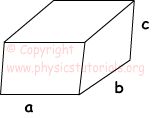distinguishing them. We show it with V and unit used in SI system is m ³.

Density of Matter

Density is the quantity of mass in a unit of volume. It is the distinguishing property of matter. Each matter has its own density. Representation of density is d; unit of it is g/cm³.

Formula of density;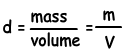Density of Mixtures

Mixtures include at least two different matters. If the matters are homogenously mixed than we called them homogenous mixtures, on the contrary if they do not mixed homogenously we called them heterogeneous mixtures.

If we have more than one substance;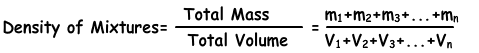Some Tricks for Density of Mixtures

1. If the volumes of the matters are equal in a given mixture then we calculate the density of mixture with following formula;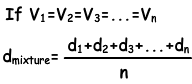2. If the masses of the matters are equal in a given mixture then we calculate the density of the mixture with following formula;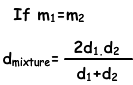Elasticity

If an external force is applied to a material, it causes deformation in molecular structure of that material. By removing this force, material turns its original shapes; we call this process as elasticity of material.

Properties of Matter Exams and Solutions

Author: